$\newcommand{\NN}{\mathbb{N}} \newcommand{\CC}{\mathbb{C}} \newcommand{\GG}{\mathbb{G}} \newcommand{\LL}{\mathbb{L}} \newcommand{\PP}{\mathbb{P}} \newcommand{\QQ}{\mathbb{Q}} \newcommand{\RR}{\mathbb{R}} \newcommand{\VV}{\mathbb{V}} \newcommand{\ZZ}{\mathbb{Z}} \newcommand{\FF}{\mathbb{F}} \newcommand{\KK}{\mathbb{K}} \newcommand{\UU}{\mathbb{U}} \newcommand{\EE}{\mathbb{E}} \newcommand{\Aa}{\mathcal{A}} \newcommand{\Bb}{\mathcal{B}} \newcommand{\Cc}{\mathcal{C}} \newcommand{\Dd}{\mathcal{D}} \newcommand{\Ee}{\mathcal{E}} \newcommand{\Ff}{\mathcal{F}} \newcommand{\Gg}{\mathcal{G}} \newcommand{\Hh}{\mathcal{H}} \newcommand{\Ii}{\mathcal{I}} \newcommand{\Jj}{\mathcal{J}} \newcommand{\Kk}{\mathcal{K}} \newcommand{\Ll}{\mathcal{L}} \newcommand{\Mm}{\mathcal{M}} \newcommand{\Nn}{\mathcal{N}} \newcommand{\Oo}{\mathcal{O}} \newcommand{\Pp}{\mathcal{P}} \newcommand{\Qq}{\mathcal{Q}} \newcommand{\Rr}{\mathcal{R}} \newcommand{\Ss}{\mathcal{S}} \newcommand{\Tt}{\mathcal{T}} \newcommand{\Uu}{\mathcal{U}} \newcommand{\Vv}{\mathcal{V}} \newcommand{\Ww}{\mathcal{W}} \newcommand{\Xx}{\mathcal{X}} \newcommand{\Yy}{\mathcal{Y}} \newcommand{\Zz}{\mathcal{Z}} \newcommand{\al}{\alpha} \newcommand{\la}{\lambda} \newcommand{\ga}{\gamma} \newcommand{\Ga}{\Gamma} \newcommand{\La}{\Lambda} \newcommand{\Si}{\Sigma} \newcommand{\si}{\sigma} \newcommand{\be}{\beta} \newcommand{\de}{\delta} \newcommand{\De}{\Delta} \renewcommand{\phi}{\varphi} \renewcommand{\th}{\theta} \newcommand{\om}{\omega} \newcommand{\Om}{\Omega} \renewcommand{\epsilon}{\varepsilon} \newcommand{\Calpha}{\mathrm{C}^\al} \newcommand{\Cbeta}{\mathrm{C}^\be} \newcommand{\Cal}{\text{C}^\al} \newcommand{\Cdeux}{\text{C}^{2}} \newcommand{\Cun}{\text{C}^{1}} \newcommand{\Calt}{\text{C}^{#1}} \newcommand{\lun}{\ell^1} \newcommand{\ldeux}{\ell^2} \newcommand{\linf}{\ell^\infty} \newcommand{\ldeuxj}{{\ldeux_j}} \newcommand{\Lun}{\text{\upshape L}^1} \newcommand{\Ldeux}{\text{\upshape L}^2} \newcommand{\Lp}{\text{\upshape L}^p} \newcommand{\Lq}{\text{\upshape L}^q} \newcommand{\Linf}{\text{\upshape L}^\infty} \newcommand{\lzero}{\ell^0} \newcommand{\lp}{\ell^p} \renewcommand{\d}{\ins{d}} \newcommand{\Grad}{\text{Grad}} \newcommand{\grad}{\text{grad}} \renewcommand{\div}{\text{div}} \newcommand{\diag}{\text{diag}} \newcommand{\pd}{ \frac{ \partial #1}{\partial #2} } \newcommand{\pdd}{ \frac{ \partial^2 #1}{\partial #2^2} } \newcommand{\dotp}{\langle #1,\,#2\rangle} \newcommand{\norm}{|\!| #1 |\!|} \newcommand{\normi}{\norm{#1}_{\infty}} \newcommand{\normu}{\norm{#1}_{1}} \newcommand{\normz}{\norm{#1}_{0}} \newcommand{\abs}{\vert #1 \vert} \newcommand{\argmin}{\text{argmin}} \newcommand{\argmax}{\text{argmax}} \newcommand{\uargmin}{\underset{#1}{\argmin}\;} \newcommand{\uargmax}{\underset{#1}{\argmax}\;} \newcommand{\umin}{\underset{#1}{\min}\;} \newcommand{\umax}{\underset{#1}{\max}\;} \newcommand{\pa}{\left( #1 \right)} \newcommand{\choice}{ \left\{ \begin{array}{l} #1 \end{array} \right. } \newcommand{\enscond}{ \left\{ #1 \;:\; #2 \right\} } \newcommand{\qandq}{ \quad \text{and} \quad } \newcommand{\qqandqq}{ \qquad \text{and} \qquad } \newcommand{\qifq}{ \quad \text{if} \quad } \newcommand{\qqifqq}{ \qquad \text{if} \qquad } \newcommand{\qwhereq}{ \quad \text{where} \quad } \newcommand{\qqwhereqq}{ \qquad \text{where} \qquad } \newcommand{\qwithq}{ \quad \text{with} \quad } \newcommand{\qqwithqq}{ \qquad \text{with} \qquad } \newcommand{\qforq}{ \quad \text{for} \quad } \newcommand{\qqforqq}{ \qquad \text{for} \qquad } \newcommand{\qqsinceqq}{ \qquad \text{since} \qquad } \newcommand{\qsinceq}{ \quad \text{since} \quad } \newcommand{\qarrq}{\quad\Longrightarrow\quad} \newcommand{\qqarrqq}{\quad\Longrightarrow\quad} \newcommand{\qiffq}{\quad\Longleftrightarrow\quad} \newcommand{\qqiffqq}{\qquad\Longleftrightarrow\qquad} \newcommand{\qsubjq}{ \quad \text{subject to} \quad } \newcommand{\qqsubjqq}{ \qquad \text{subject to} \qquad }$

Dictionary Learning

# Dictionary Learning

Instead of using a fixed data representation such as wavelets or Fourier, one can learn the representation (the dictionary) to optimize the sparsity of the representation for a large class of exemplar.

## Installing toolboxes and setting up the path.

You need to download the following files: signal toolbox and general toolbox.

You need to unzip these toolboxes in your working directory, so that you have toolbox_signal and toolbox_general in your directory.

For Scilab user: you must replace the Matlab comment '%' by its Scilab counterpart '//'.

Recommandation: You should create a text file named for instance numericaltour.sce (in Scilab) or numericaltour.m (in Matlab) to write all the Scilab/Matlab command you want to execute. Then, simply run exec('numericaltour.sce'); (in Scilab) or numericaltour; (in Matlab) to run the commands.

Execute this line only if you are using Matlab.

getd = @(p)path(p,path); % scilab users must *not* execute this


Then you can add the toolboxes to the path.

getd('toolbox_signal/');
getd('toolbox_general/');


## Dictionary Learning as a Non-convex Optimization Problem

Given a set $$Y = (y_j)_{j=1}^m \in \RR^{n \times m}$$ of $$m$$ signals $$y_j \in \RR^m$$, dictionary learning aims at finding the best dictionary $$D=(d_i)_{i=1}^p$$ of $$p$$ atoms $$d_i \in \RR^n$$ to sparse code all the data.

In this numerical tour, we consider an application to image denoising, so that each $$y_j \in \RR^n$$ is a patch of size $$n=w \times w$$ extracted from the noisy image.

The idea of learning dictionaries to sparse code image patch was first proposed in:

Olshausen BA, and Field DJ., Emergence of Simple-Cell Receptive Field Properties by Learning a Sparse Code for Natural Images. Nature, 381: 607-609, 1996.

The sparse coding of a single data $$y=y_j$$ for some $$j=1,\ldots,m$$ is obtained by minimizing a $$\ell^0$$ constrained optimization $\umin{ \norm{x}_0 \leq k } \frac{1}{2}\norm{y-Dx}^2 .$ where the $$\ell^0$$ pseudo-norm of $$x \in \RR^p$$ is $\norm{x}_0 = \abs{\enscond{i}{x(i) \neq 0}}.$

The parameter $$k>0$$ controls the amount of sparsity.

Dictionary learning performs an optimization both on the dictionary $$D$$ and the set of coefficients $$X = (x_j)_{j=1}^m \in \RR^{p \times m}$$ where, for $$j=1,\ldots,m$$, $$x_j$$ is the set of coefficients of the data $$y_j$$. This joint optimization reads $\umin{ D \in \Dd, X \in \Xx_k } E(X,D) = \frac{1}{2}\norm{Y-DX}^2 = \frac{1}{2} \sum_{j=1}^m \norm{y_j - D x_j}^2.$

The constraint set on $$D$$ reads $\Dd = \enscond{D \in \RR^{n \times p} }{ \forall i=1,\ldots,p, \quad \norm{D_{\cdot,i}} \leq 1 },$ (the columns of the dictionary are unit normalized). The sparsity constraint set on $$X$$ reads $\Xx_k = \enscond{X \in \RR^{p \times m}}{ \forall j, \: \norm{X_{\cdot,j}}_0 \leq k }.$

We propose to use a block-coordinate descent method to minimize $$E$$: $X^{(\ell+1)} \in \uargmin{X \in \Xx_k} E(X,D^{(\ell)}),$ $D^{(\ell+1)} \in \uargmin{D \in \Dd} E(X^{(\ell+1)},D).$

One can show the convergence of this minimization scheme, see for instance

P. Tseng, Convergence of Block Coordinate Descent Method for Nondifferentiable Minimization, J. Optim. Theory Appl., 109, 2001, 475-494.

We now define the parameter of the problem.

Width $$w$$ of the patches.

if not(exist('w'))
w = 10;
end


Dimension $$n= w \times w$$ of the data to be sparse coded.

n = w*w;


Number of atoms $$p$$ in the dictionary.

p = 2*n;


Number $$m$$ of patches used for the training.

m = 20*p;


Target sparsity $$k$$.

k = 4;


## Patch Extraction

Since the learning is computationnaly intensive, one can only apply it to small patches extracted from an image.

if not(exist('f'))
f = rescale( crop(load_image('barb'),256) );
end
n0 = size(f,1);


Display the input image.

clf;
imageplot(clamp(f));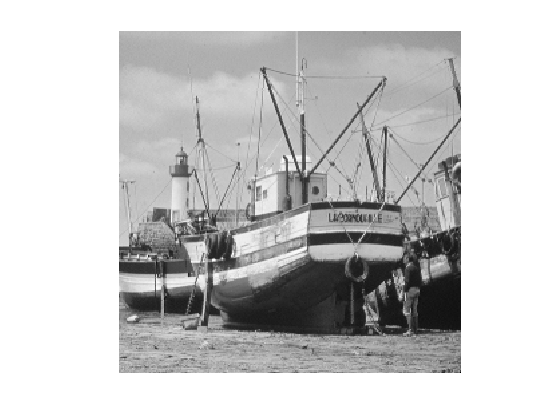Random patch location.

q = 3*m;
x = floor( rand(1,1,q)*(n0-w) )+1;
y = floor( rand(1,1,q)*(n0-w) )+1;


Extract lots of patches $$y_j \in \RR^n$$, and store them in a matrix $$Y=(y_j)_{j=1}^m$$.

[dY,dX] = meshgrid(0:w-1,0:w-1);
Xp = repmat(dX,[1 1 q]) + repmat(x, [w w 1]);
Yp = repmat(dY,[1 1 q]) + repmat(y, [w w 1]);
Y = f(Xp+(Yp-1)*n0);
Y = reshape(Y, [n q]);


We remove the mean, since we are going to learn a dictionary of zero-mean and unit norm atom.

Y = Y - repmat( mean(Y), [n 1] );


Only keep those with largest energy.

[tmp,I] = sort(sum(Y.^2), 'descend');
Y = Y(:,I(1:m));


We consider a dictionary $$D \in \RR^{n \times p}$$ of $$p \geq n$$ atoms in $$\RR^n$$. The initial dictionary $$D$$ is computed by a random selection of patches, and we normalize them to be unit-norm.

ProjC = @(D)D ./ repmat( sqrt(sum(D.^2)), [w^2, 1] );
sel = randperm(m); sel = sel(1:p);
D0 = ProjC( Y(:,sel) );
D = D0;


Display the initial dictionary.

clf;
plot_dictionnary(D, [], [8 12]);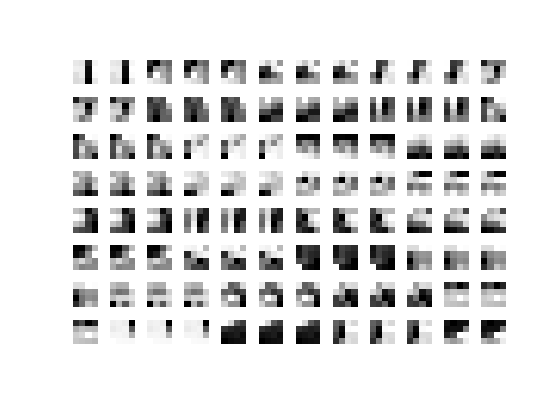## Update of the Coefficients $$X$$

The optimization on the coefficients $$X$$ requires, for each $$y_j = Y_{\cdot,j}$$ to compute $$x_j = X_{\cdot,j}$$ that solves $\umin{ \norm{x_j}_0 \leq k } \frac{1}{2} \norm{y-D x_j}^2.$

This is a non-smooth and non-convex minimization, that can be shown to be NP-hard. A heuristic to solve this method is to compute a stationary point of the energy using the Foward-Backward iterative scheme (projected gradient descent): $x_j \leftarrow \text{Proj}_{\Xx_k}\pa{ x_j - \tau D^* ( D x_j - y ) } \qwhereq \tau < \frac{2}{\norm{D D^*}}.$

Denoting $$\abs{\bar x(1)} \leq \ldots \leq \abs{\bar x(n)}$$ the ordered magnitudes of a vector $$x \in \RR^n$$, the orthogonal projector on $$\Xx_k$$ reads $$z = \text{Proj}_{\Xx_k}(x)$$ with $\forall i=1,\ldots,n, \quad z(i) = \choice{ x(i) \qifq \abs{x(i)} \geq \abs{\bar x(k)}, \\ z(i) = 0 \quad \text{otherwise}. }$

select = @(A,k)repmat(A(k,:), [size(A,1) 1]);
ProjX = @(X,k)X .* (abs(X) >= select(sort(abs(X), 'descend'),k));


Exercice 1: (check the solution) Perform the iterative hard thresholding, and display the decay of the energy $$J(x_j) = \norm{y_j-D x_j}^2$$ for several $$j$$. Remark: note that the iteration can be performed in parallel on all $$x_j$$.

exo1;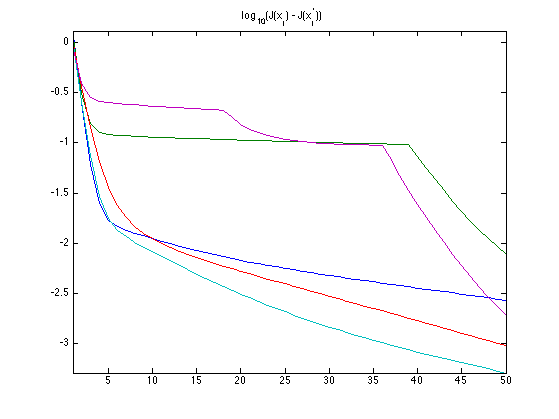## Update the Dictionary $$D$$

Once the sparse coefficients $$X$$ have been computed, one can udpate the dictionary. This is achieve by performing the minimization $\umin{D \in \Dd} \frac{1}{2}\norm{Y-D X}^2.$

One can perform this minimization with a projected gradient descent $D \leftarrow \text{Proj}_{\Cc}\pa{ D - \tau (DX - Y)X^* }$ where $$\tau < 2/\norm{XX^*}.$$

Note that the orthogonal projector $$\text{Proj}_{\Cc}$$ is implemented in the function ProjC already defined.

Exercice 2: (check the solution) Perform this gradient descent, and monitor the decay of the energy.

exo2;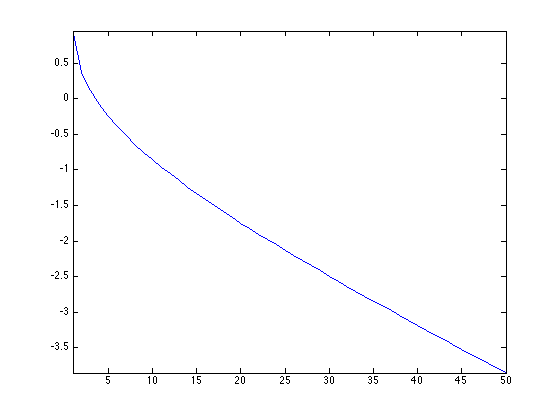Exercice 3: (check the solution) Perform the dictionary learning by iterating between sparse coding and dictionary update.

exo3;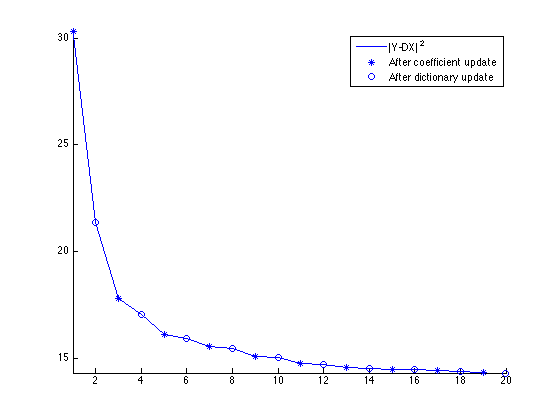Display the dictionary.

clf;
plot_dictionnary(D,X, [8 12]);

Index exceeds matrix dimensions.

Error in plot_dictionnary (line 69)
vmax = max( max( abs( D(:,I(1:prod(nb))) ) ) );

Error in index (line 256)
plot_dictionnary(D,X, [8 12]);